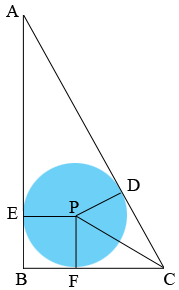SEARCH HOMEMath Central Quandaries & QueriesQuestion from Kamerson: i have been given a problem with a 30-60-90 triangle and a circle inscribed with a radius of 2 and was told to find the perimeter of the triangleHi Kamerson,

You can solve this problem using two facts.

First for a 30-6--90 triangle the lengths of the sides are in the ratio $1:2:\sqrt{3}.$

For the second fact I need to draw a diagram.P is the center of the circle and D, E and F are points of tangency. The fact I see is that triangles PFC and DPC are congruent.

Can you solve the problem now?

PennyMath Central is supported by the University of Regina and The Pacific Institute for the Mathematical Sciences.